alonsoformula In SpanishWhat are they?  |  How are they named? If we know the formula  | If we know the name  |  Examples  |  Exercises

What are they?

They are compounds formed by a nonmetal and oxygen, in which the oxygen has an oxidation number –2.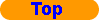How are they named?

For its nomenclature we use

Stoichiometric nomenclature: it is formed by two words. The first word is the name of the nonmetal with a Greek prefix that shows us the number of atoms of nonmetal, the second word is the name "oxide" with a Greek prefix that shows us the number of atoms of oxygen.

The Greek prefixes are:

 1 2 3 4 5 6 7 8 9 10 11 12 mono- di- tri- tetra- penta- hexa- hepta- octa- nona- deca- undeca- dodeca-

Prefix-NONMETAL prefix-oxide

The prefix mono- is used only before "oxide" and when the ratio is 1:1.

The IUPAC also accepts Stock´s nomenclature for these oxides, though it is better to use the stoichiometric nomenclature when all the atoms are nonmetals and Stock´s nomenclature when there are metal and non metal atoms.If we know the formula

In the formula: Name of the nonmetallic element with a Greek prefix that shows us the number of atoms, followed by oxide with a Greek prefix that shows us the number of atoms of oxygen.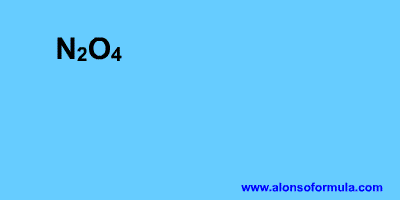If we know the name

In the name: The first word says the first element in the formula, the prefix indicates the subscript of the element in the formula, "oxide" indicates that the second element is the oxygen, and the prefix indicates the subscript of the oxygen in the formula.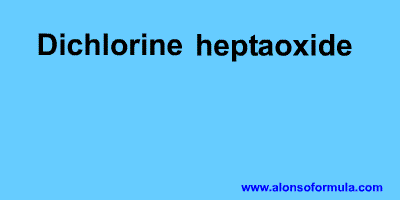Examples

 Stoichiometric nomenclature N2O Dinitrogen oxide NO Nitrogen monoxide N2O3 Dinitrogen trioxide CO Carbon monoxide CO2 Carbon dioxideExercises

In Formulae you have an exercise to write the names of these substances and to check your results. You also have the answer to the exercise.

In Names you have an exercise to write the formulae for these substances and to check your results. You have to introduce the formulae without subscripts, for example for water = H2O. You also have the answer to the exercise.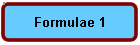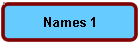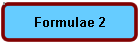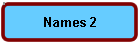Home | Introduction | Oxidation number | Mechanics | Kind of substances | Elementary substances | Metal oxides | Nonmetal oxides | Metal-nonmetal compounds | Nonmetal-nonmetal compounds | Metal hydrides | Hydracids | H with nonmetal | Hydroxides | Oxyacids | Oxysalts | Acid salts | Exercises | Periodic Table | Links
Author: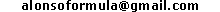WWW.ALONSOFORMULA.COM Formulación Inorgánica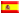Formulación OrgánicaFormulación Inorgánica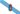Formulación OrgánicaFormulació InorgánicaFormulació OrgánicaEzorganikoaren Formulazioa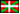Nomenclature of Inorganic Q.Física y Química de ESOFísica e Química de ESOFQ de 1º de BachilleratoFQ de 1º de BacharelatoQuímica de 2º de BachilleratoPrácticas de Química